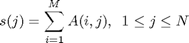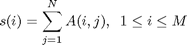# “DIM” terminology

I've been bothered recently by a MATLAB terminology question: How should we describe the dim argument in functions such as sum? The documentation for sum says:

  B = sum(A,dim) sums along the dimension of A specified by
scalar dim. The dim input is an integer value from 1 to N,
where N is the number of dimensions in A. Set dim to 1 to
compute the sum of each column, 2 to sum rows, etc.

For example:

a = [1 2 3; 4 5 6; 7 8 9]
a =

1     2     3
4     5     6
7     8     9


sum(a, 1)
ans =

12    15    18


sum(a, 2)
ans =

6
15
24



For some people this terminology seems to be quite confusing. I started thinking about this when I saw a user question about it recently. In that question, the mental model leading to confusion seems to be this: dimension 1 is the row dimension, so sum(a,1) should sum each row, but MATLAB sums each column.

This a hard mental model to shake, apparently, and it makes me wonder if the phrase "along the dimension" isn't clear enough.

When you look at equations instead of ambiguous prose, it's easier to see the logic of the dim argument. Consider, for example, an M-by-N matrix A. sum(A,1) is doing this:The variable of summation, i, is the first subscript of A.

sum(A,2) is doing this:The variable of summation, j, is the second subscript of A.

This pattern extends nicely to higher dimensions.

So, dear readers, can you suggest a better way to document the meaning of the dim argument? Or should we just leave it be?

Published with MATLAB® 7.10

|